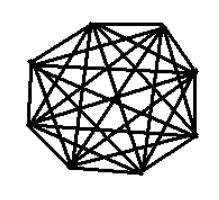## 7.201 Isomorphic Graphs

• Isomorphism

• Two graphs $G_1$ and $G_2$ are isomorphic if there is a bijection (invertible function) $f: G_1 \rightarrow G_2$ that preserves adjacency and non-adjacency.

• if $uv$ is in $E(G_1)$ then $f(u)f(v)$ is in $E(G_2)$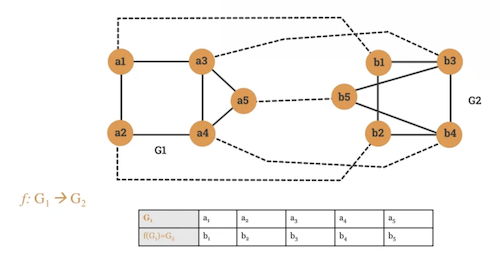• Two graphs with different degree sequences can't be ismorphic.

• Properties of isomorphic graphs
• 2 graphs with different degree sequence can't be isomorphic.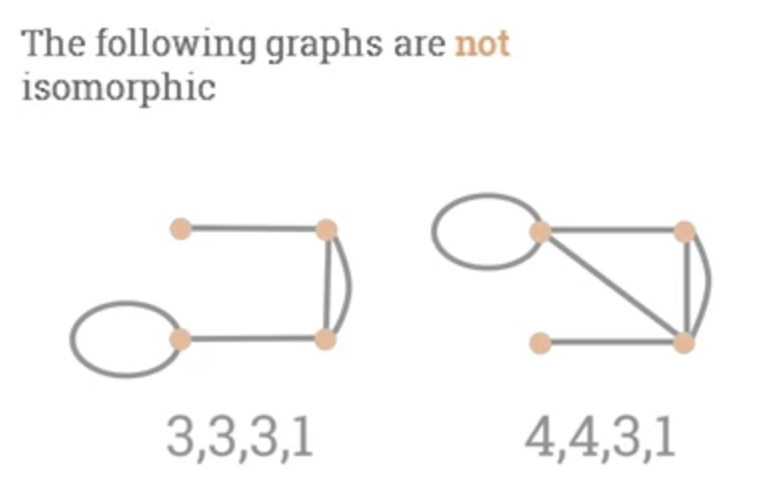• 2 graphs with the same degree sequence may not be isomorphic.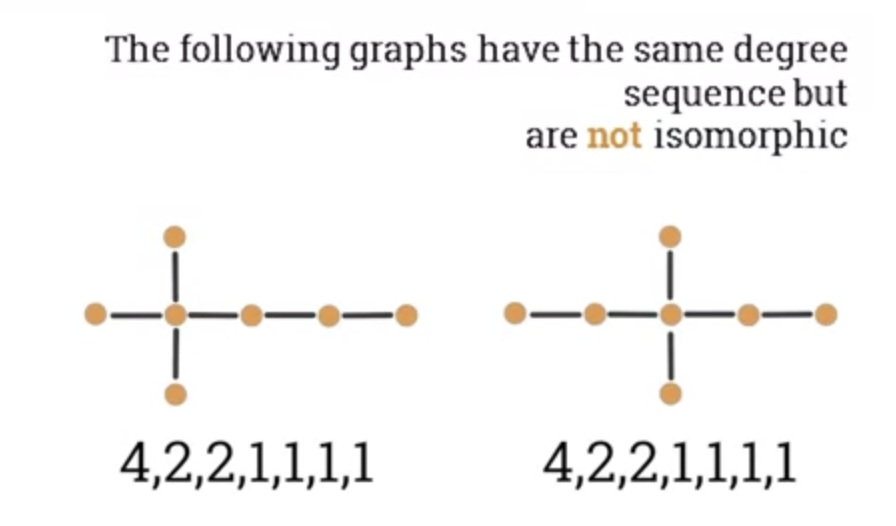## 7.203 Bipartite graphs

• Bipartite Graph

• A graph $G(V, E)$ is called a bi-partite graph.
• If the set of vertices V can be partitioned in 2 no-empty disjoint sets $V_1$ and $V_2$ in such a way that each edge $e$ in $G$ has one endpoint in $V_1$ and another endpoint in $V_2$.
• Example: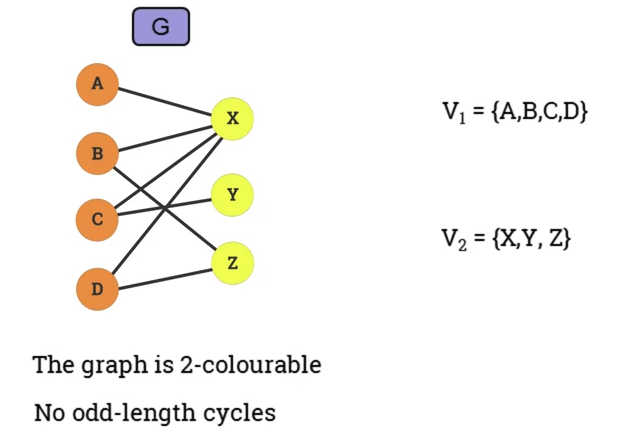• Graph is 2-colourable.
• No odd-length cycles.
• Matching
• A set of pairwise non-adjacent edges, none of which are loops.
• ie no 2 edges share a common endpoint.
• A vertex is matched (or saturated) if it is an endpoint of one of the edges in the matching.

• Otherwise the vertex is unmatched.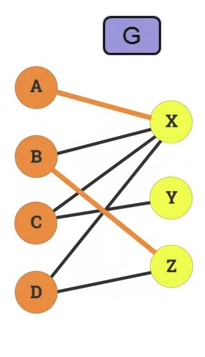• Maximum matching

• A maximum matching is a matching of maximum size so that if any edge is added, it's no longer matching.
• In a bitpartite graph, there can be multiple maximum matching.
• The Hopcroft-Kaft Algorithm

• An algorithm for solving the maximum matching problem in a bipartite graph.
• Concepts:
• Augmenting path: starts on a free node and alternate between unmatched unmatched edges ending on a free node
• "augments the cardinality of the current machine"
• Traverses the graph level by level
• Depth-first search:
• Traverses graph all the way to a leaf before starting another path.
• Example: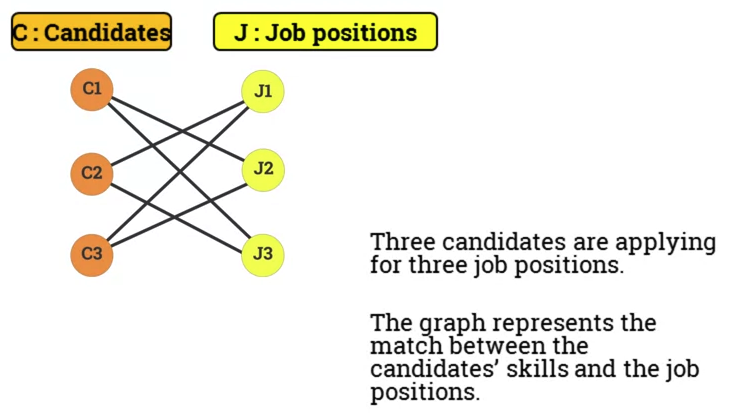• Pseduo code:
• Initialise M = {}
• While there exists an Augmenting Path p
1. Use BFS to build layers that terminate at free vertices.
1. Start at the free vertices in C, use DFS.
• Return M

## 7.205 The adjacency matrix of a graph

• Adjaceny List of a graph.

• So far a graph has been represented by a set of vertices and a set of edges.
• Adjaceny list of a graph G is a list of all vertices in G and their corresponding individual adjacent vertices.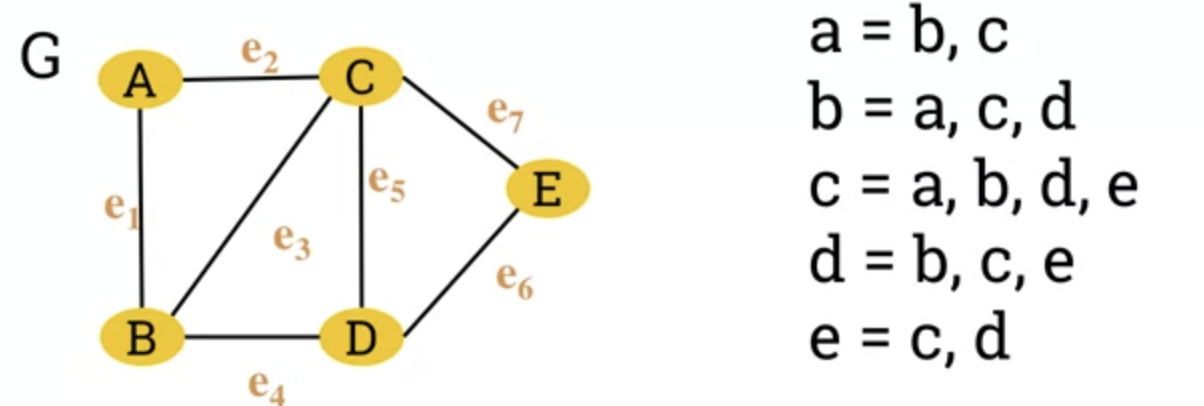• Adjacent Matrix of a graph.

• A graph can also be represented by its adjacency matrix.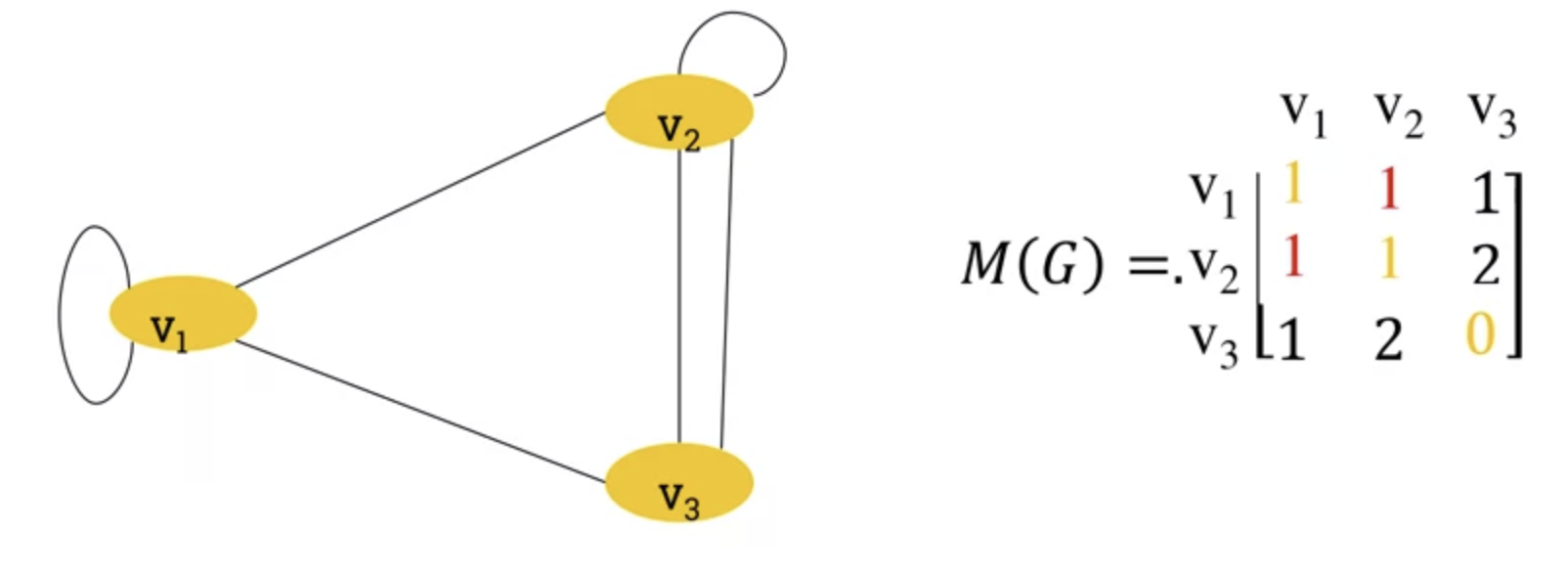• Apparent from the loops, ever other edge is represented twice (ie v1 -> v3 and v3->v1)
• So we can multiply the diagonal by 2 to represent loops consistently.
• Properties of the adjaceny matrix.
• Adjaceny matrix of an undirected graph is symmetric.
• Number of edges in undirected graph equals half the sum of all elements ($\mathbf{m_ij}$) of its corresponding adjaceny matrix.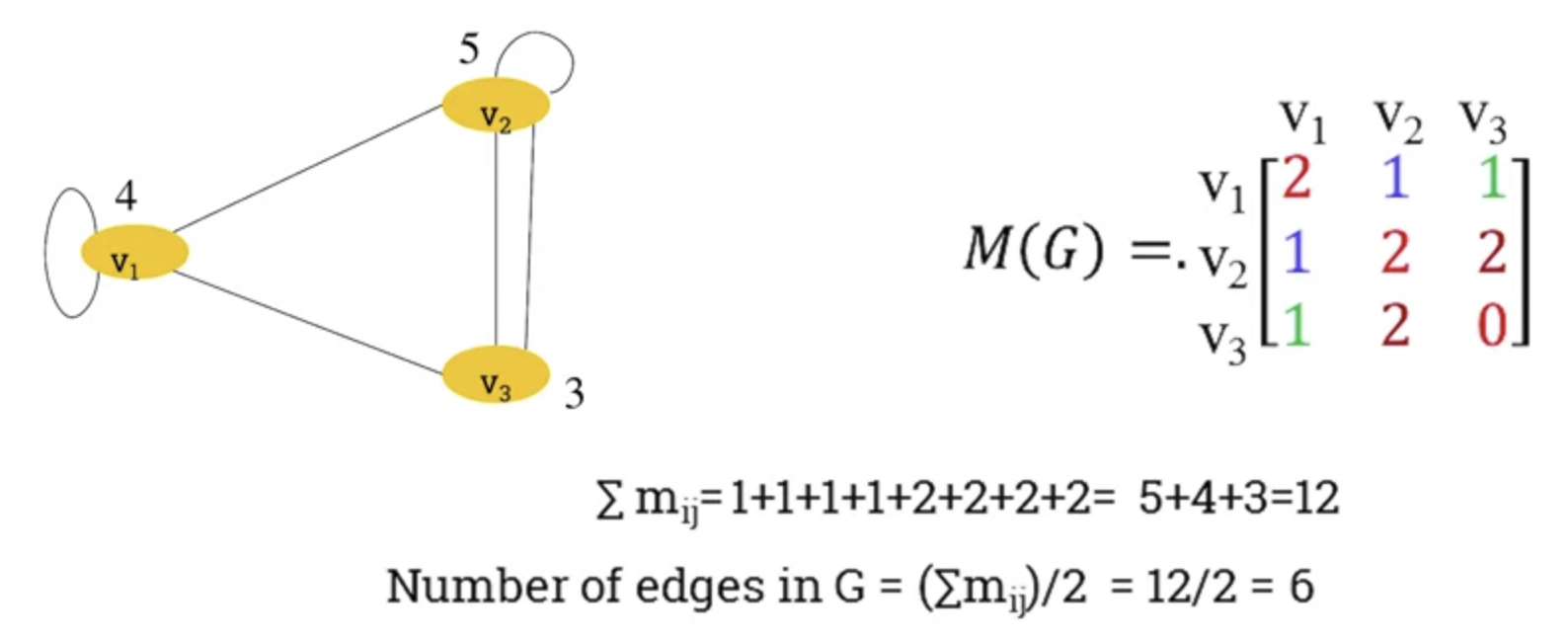• In a directed graph, the adjaceny matrix only counts an edge pointing in a certain direction once.

## 7.207 Dijkstra's algorithm

• Weighted Graphs

• A weighted graph is a graph where each edge is assigned a numerical weight.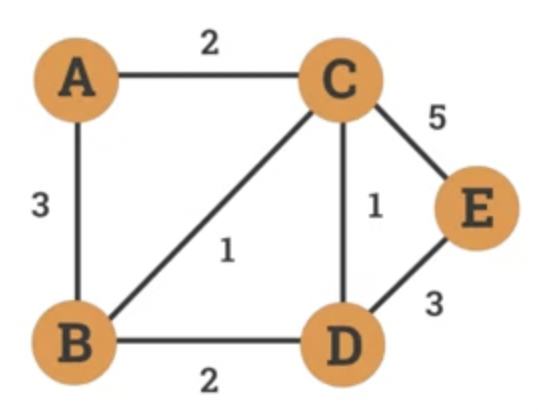• Can be used to model:
• Distance between cities.
• Response time in communication network.
• Cost of transaction.
• Dijkstra's algorithm
• An algorithm designed by Edsger W. Dijkstra in 1956.
• Find shortest path between nodes in weighted graph.
• Example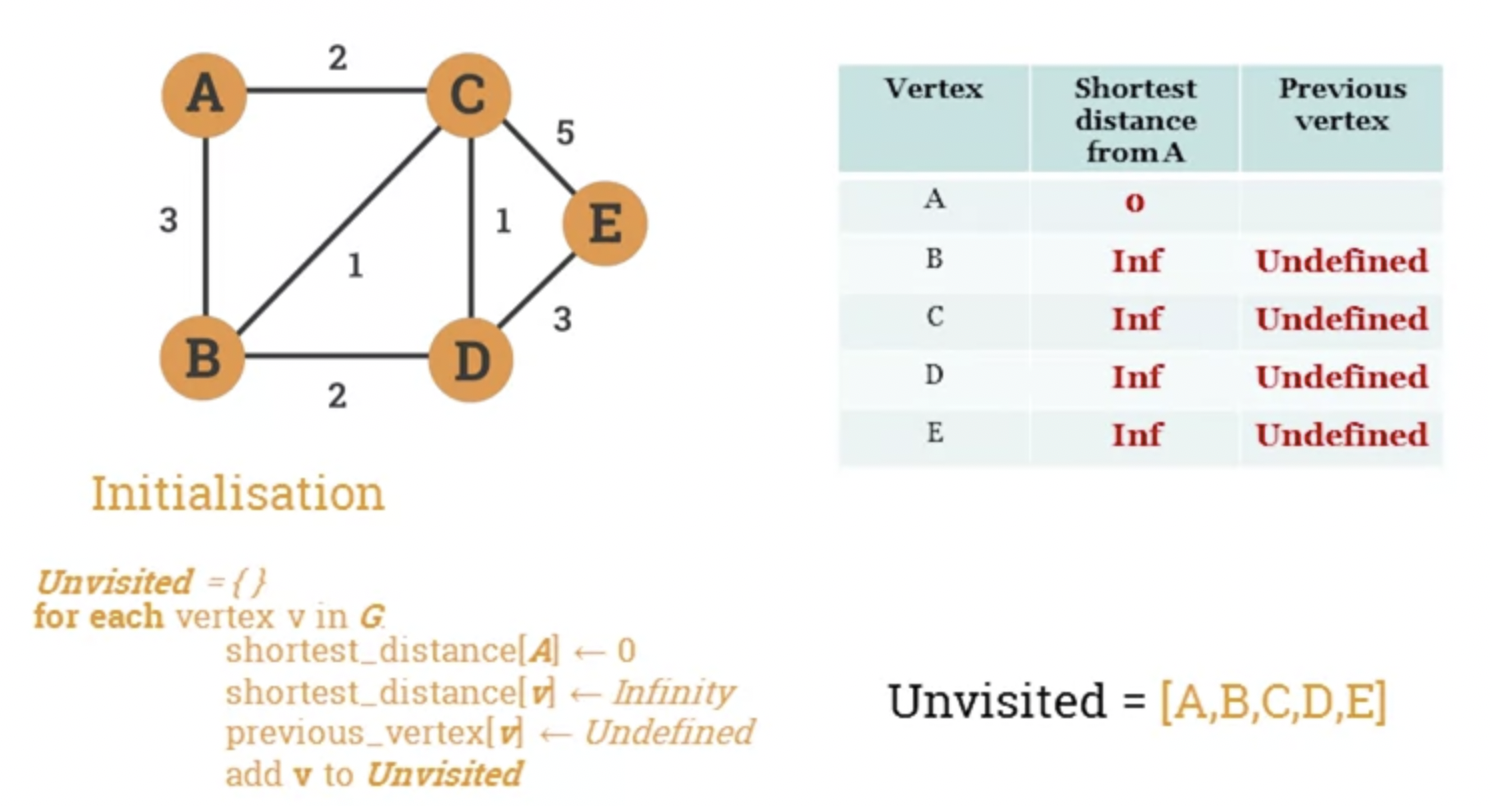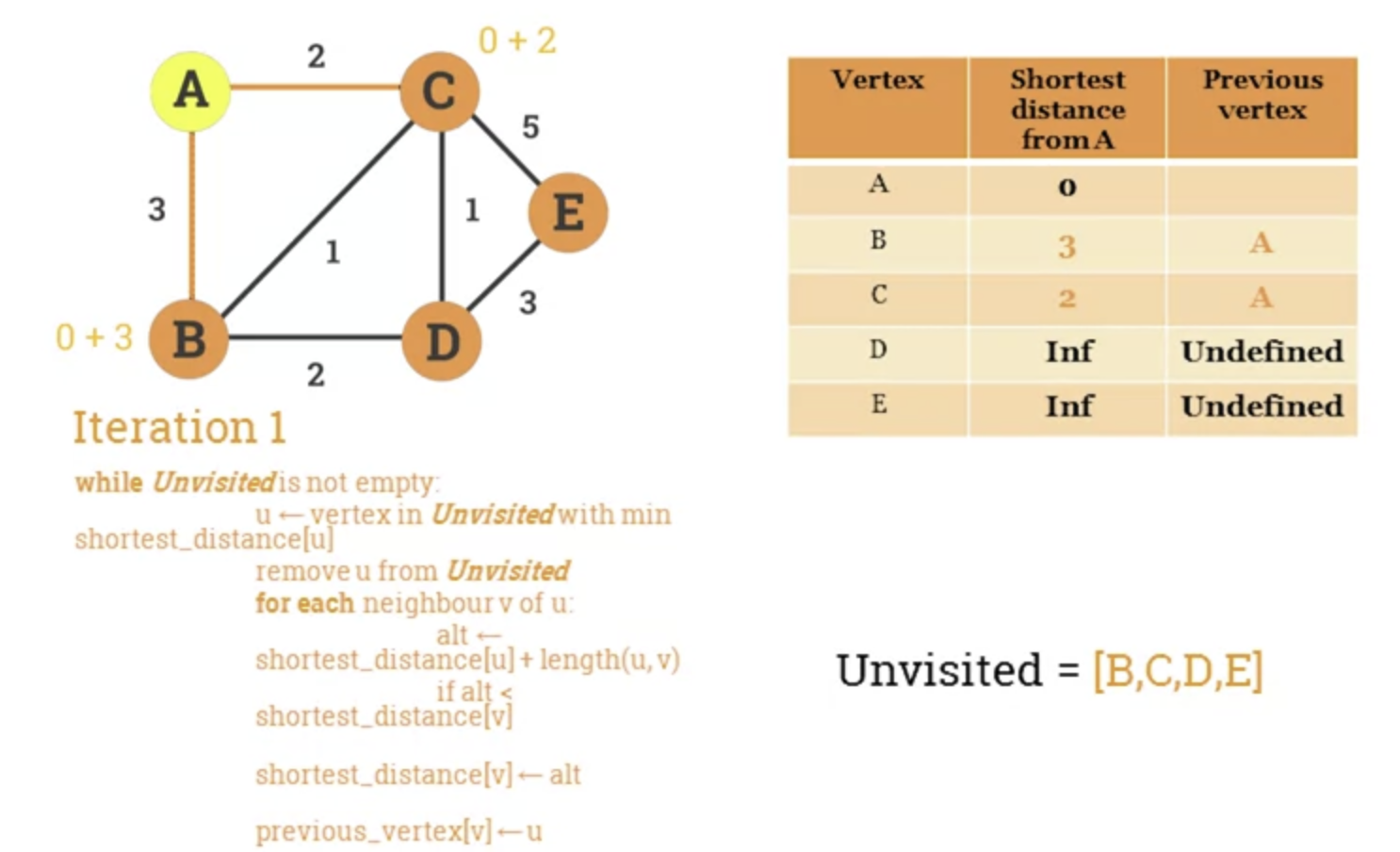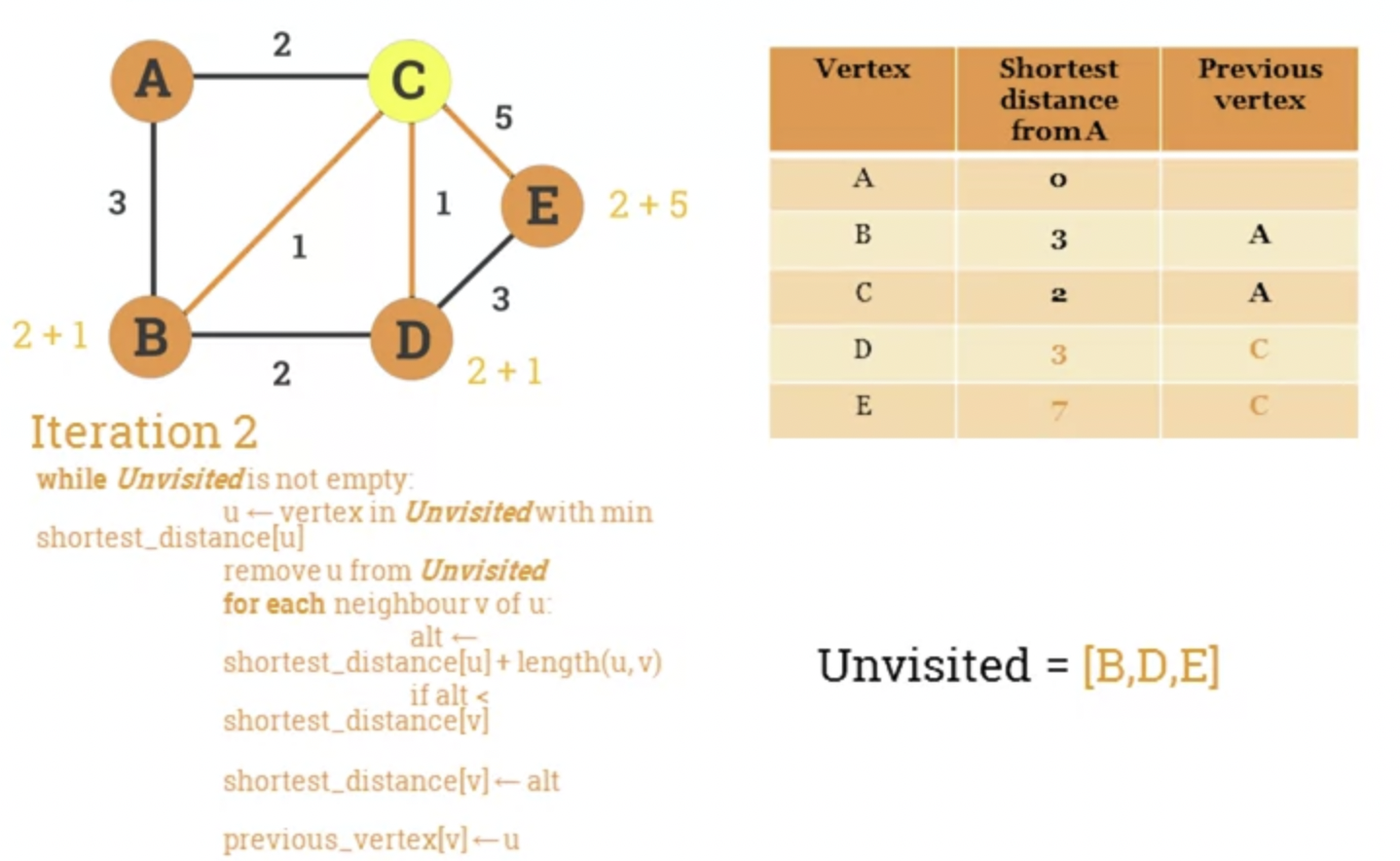• Algorithm's pseudocode.

• Let G be a graph and s a source vertex.
• The following pseudocode calculates the shortest distance and previous vertex from s to every other node in the graph.
Unvisited = {}
for each vertex v in G:
shortest_distanced[v] = Infinity
previous_vertex[v] = Undefined
shortest_distance[s] = 0
while Unvisited is not empty:
u = vertex in Unvisited with min shortest_distance[u]
remove u from Unvisited
for each neighbour v of u:
alt = shortest_distance[u] + length(u, v)
if alt < shortest_distance[v]:
shortest_distance[v] = alt
previous_vertex[v] =u
return shortest_distance, previous_vertex


## Problem Sheet

### Question 1

Given the following graph $G := (V, E)$

$V = \{ v1, v2, v3, v4, v5, v6 \}$ $E = \{ \{v1, v2\}, \{v2, v5\}, \{v5, v3\}, \{v3, v4\}, \{v2, v4\}, \{u6, u6\} \}$

1. Draw the graph G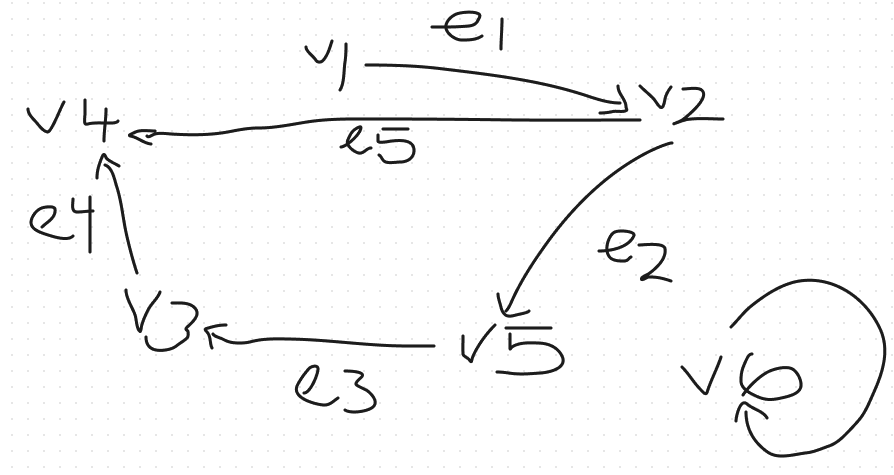1. List the set of vertices adjacent to v2

v1, v4, v5

1. List the set of edges incident with v3.

(v3, v4), (v5, v3)

1. Give an example of a path of length 3 starting at the vertex v2 and ending at v5.

v2, v4, v3, v5

1. Give an example of a cycle length 4.

v2, v4, v3, v5, v2

## Question 2

Given the following graph: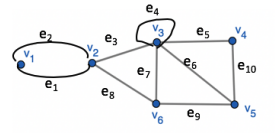Determine which of the walks are trails, paths or circuits.

1. v1 e1 v2 e3 v3 e4 v3 e5 v4

2. Is a trail as no edge is repeated.

3. Is NOT a path as v3 is repeated.
4. Is NOT a circuit as it is not closed.

5. e1 e3 e5 e5 e6

6. Is NOT a trail as e5 is repeated.

7. v2 v3 v4 v5 v3 v6 v2

v2 e3 v3 e5 v4 e10 v5 e5 v3 e7 v6 e8 v2

• Is a trail as no edge is repeated.
• Is NOT a path as v3 is repeated.
• Is a circuit as it's a closed trail.

• v2 v3 v4 v5 v6 v2

v2 e3 v3 e5 v4 e10 v5 e9 v6 e8 v2

• Is a trail as no edge repeated.
• Is NOT a path as v2 is repeated
• Is a circuit from v2 to v2

• v1 e1 v2 e1 v1

• Not a trail as e1 is repeated.

## Question 3

Which of the following undirected graphs have a Euler circuit? Which of those that do not have an Euler circuit have a Euler path?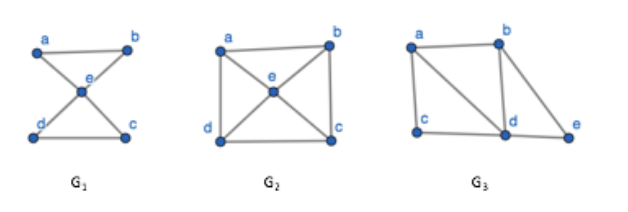• Euler path
• A graph that has a path that uses each edge of the graph exactly once. If the path exists, the graph is considered traversable.
• Euler Circuit

• A graph with a circuit (starts and ends on same vertice) containing all edges.
• If a graph has a Euler circuit, then every vertex of the graph has a positive even integer degree.
• G1 has a Euler Circuit. Is has a closed trail that uses each edge exactly

ab, bd, dc, ce, ea

1. G2 does not have Euler Circuit. It does not have a Euler path.
2. G3 does not have a Euler Circuit. However, it does have a Euler path.

ab, bd, da, ac, cd, de, eb

## Question 4

Which of the following directed graphs has an Euler circuit? Which of those that do not have a Euler path?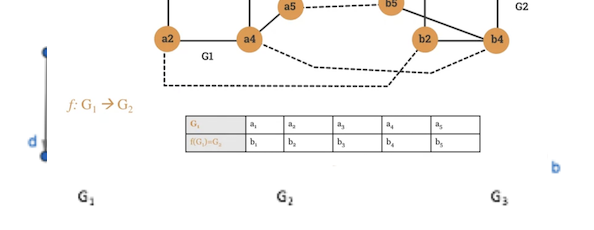1. Does not have a Euler circuit, or path.
2. Does have a Euler circuit

ag, gc, cb, bg, ge, ed, df, fa

1. Does not have a Euler circuit, but does have a path.

ca, ab, bc, cd, db

## Question 5

In each of the following either construct a graph with the specified properties or say why it is not possible to do it.

1. A graph with degree sequence 4, 3, 3, 1

The degree sequence here adds to 11. The sum of a degree sequence must be even.

1. A simple graph with degree sequence 4, 3, 3, 2, 2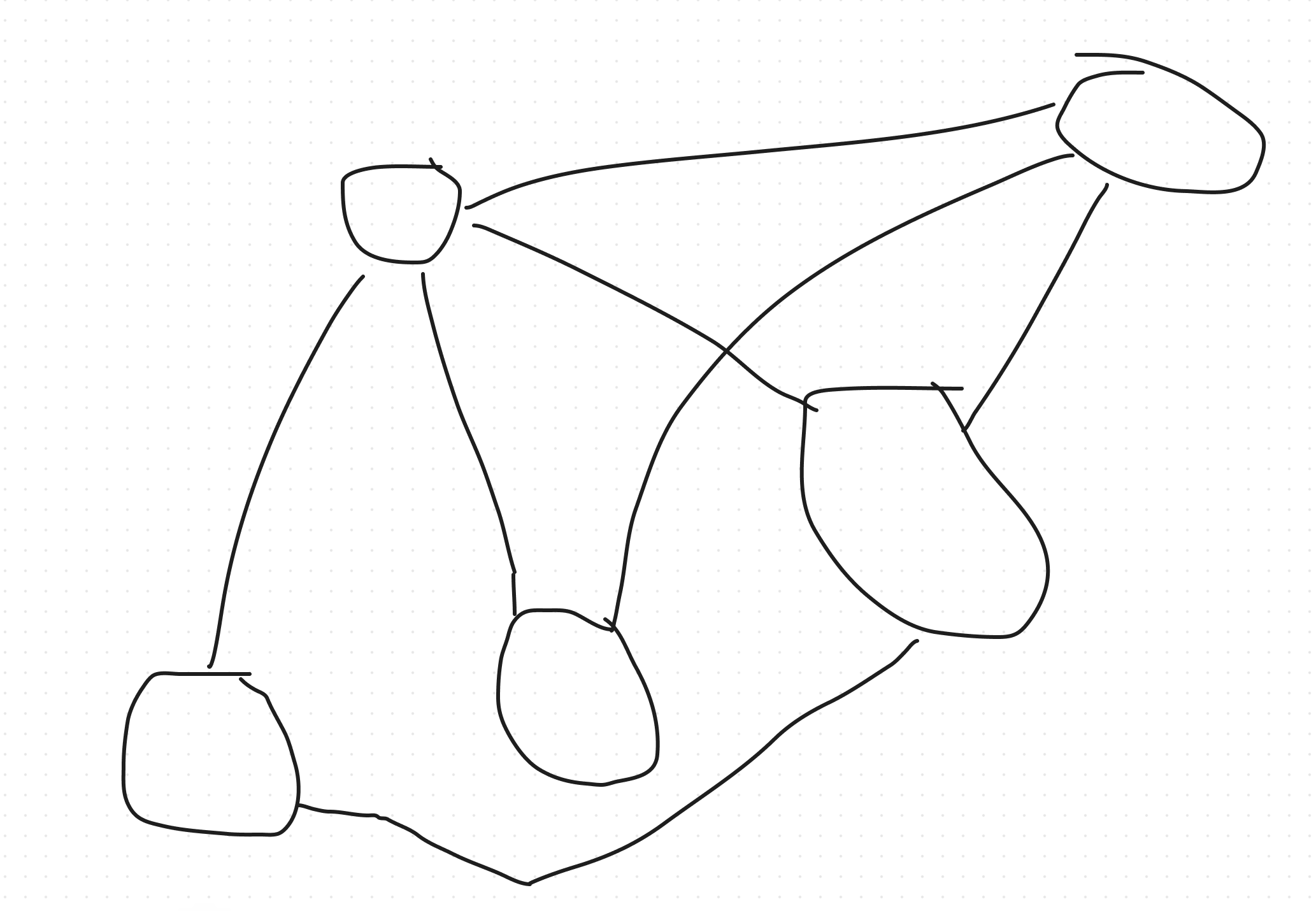1. A simple 3 regular graph with 6 vertices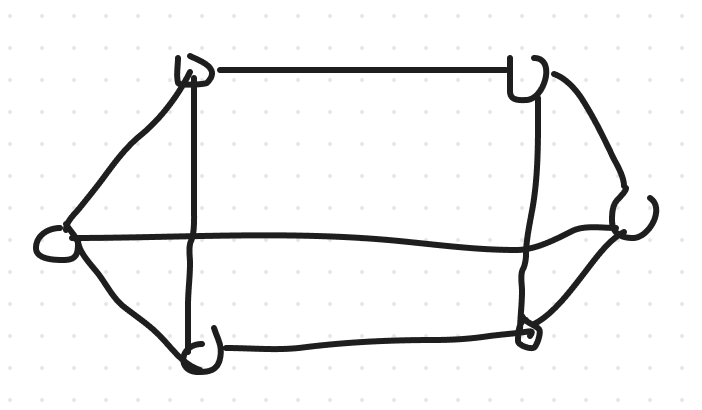A

## Question 6

In a group of 25 people, is it possible to each shake hands with exacty 3 other people.

I think it's not, as in order to have a degree sequence of 3 with 25 vertices, you would end up with an odd sum.

## Question 7

Find a Hamiltonian circuit in the following graph: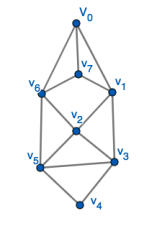## Question 8

Given the following directed graph: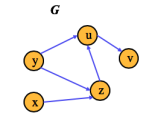Find the transitive closure, G*, of the graph G.

To find the Transitive Closure of G, we need to add missing edges if there are any. It's constructed like this:

1. Take the starting point as the graph G.
2. Check if there is a directed path between and 2 vertices of G. For example, a directed path from vertex u to v.
3. Then add a direct path if it's not already in the graph.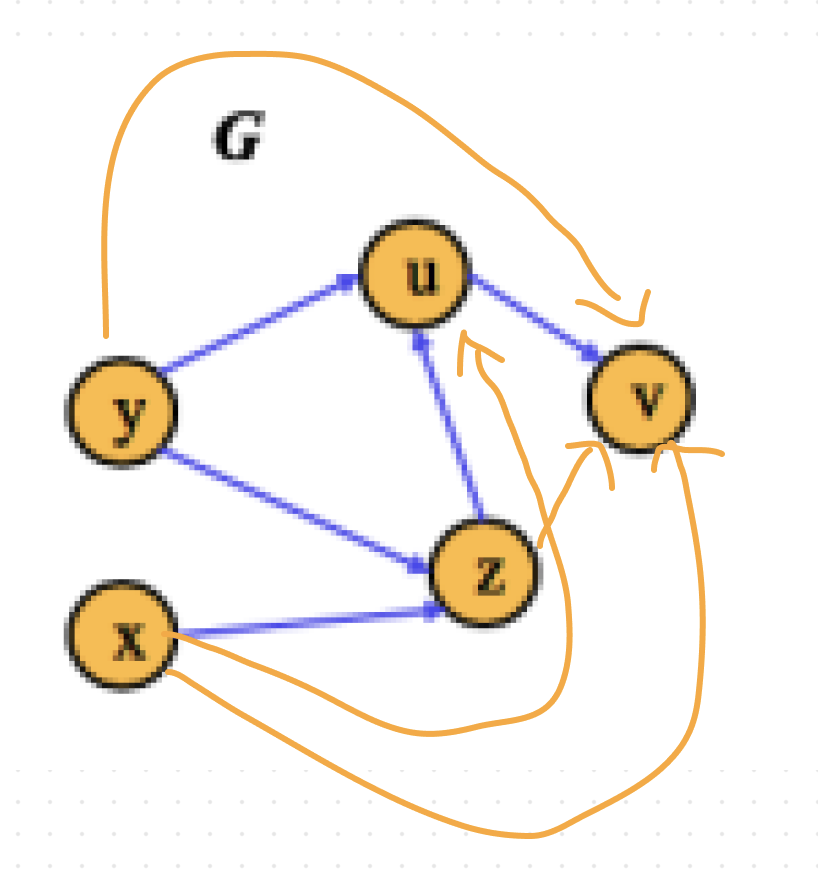## Question 9

Suppose that 7 sites are connected in a network. The number of other sites to which each site has a direct connection is given by the following sequence:

1, 2, 2, 3, 3, 4, 7

1. This might describe some kind of remote office, with a head office with direct connectivity to each suboffice, then 3 countries with 1-3 sites. Each country has connectivity to other offices and head office.
2. It has 7 vertices.
3. The sum of degrees is twice the number of edges: $\frac{7 + 4 + 3 + 3 + 2 + 2 +1}{2} = 11$
4. It is impossible to construct a Simple Graph, as there are n vertices and for a simple graph, the degree of each vertices is at most n-1 or 6. We have a vertice with 7 connections.
5. It is impossible to construct a network with 9 sites, with 5 connections as that would result in $9 \ x \ 5 = 45$ which is an odd number. A degree sequence must be even.

## Question 10

1. What is a Complete Graph?

1. What is the degree of each vertex of the complete graph $K_8$? Calculate the number of edges in $K_8$. Draw $K_8$